Next: Equilibrium of a Rotating Up: Hydrostatics Previous: Curve of Buoyancy

# Rotational Hydrostatics

Consider the equilibrium of an incompressible fluid that is uniformly rotating at a fixed angular velocityin some inertial frame of reference. Of course, such a fluid appears stationary in a non-inertial co-rotating reference frame. Moreover, according to standard Newtonian dynamics (Fitzpatrick 2012), the force balance equation for the fluid in the co-rotating frame takes the form (cf., Section 2.2)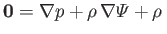(2.82)

where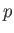is the static fluid pressure,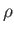the mass density,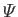the gravitational potential energy per unit mass, and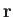a position vector (measured with respect to an origin that lies on the axis of rotation). The final term on the right-hand side of the previous equation represents the fictitious centrifugal force density. Without loss of generality, we can assume that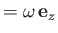. It follows that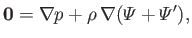(2.83)

where(2.84)

is the so-called centrifugal potential. Recall, incidentally, thatis a uniform constant in an incompressible fluid.

As an example, consider the equilibrium of a body of water, located on the Earth's surface, that is uniformly rotating about a vertical axis at the fixed angular velocity. It is convenient to adopt cylindrical coordinates (see Section C.3),,,, whose symmetry axis coincides with the axis of rotation. Letincrease upward. It follows thatand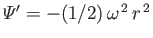. Assuming that the pressure distribution is axisymmetric, so that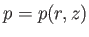, the force balance equation, (2.83), reduces to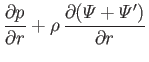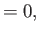(2.85)(2.86)

or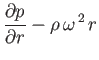(2.87)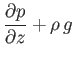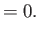(2.88)

The previous two equations can be integrated to give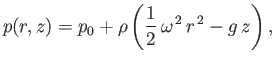(2.89)

where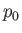is a constant. Thus, constant pressure surfaces in a uniformly rotating body of water take the form of paraboloids of revolution about the rotation axis. Suppose thatrepresents atmospheric pressure. In this case, the surface of the water is the locus of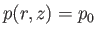: that is, it is the constant pressure surface whose pressure matches that of the atmosphere. It follows that the surface of the water is the paraboloid of revolution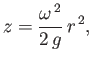(2.90)

whereis the perpendicular distance from the axis of rotation, and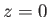the on-axis height of the surface.

According to the analysis of Section 2.3, the buoyancy force acting on any co-rotating solid body, which is wholly or partially immersed in the water, is the same as that which would maintain the mass of water displaced by the body in relative equilibrium. In the case of a floating body, this mass is limited by the continuation of the water's curved surface through the body. Let points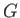andrepresent the centers of gravity and buoyancy, respectively, of the body. Of course, the latter point is simply the center of gravity of the displaced water. Suppose thatandare located perpendicular distances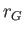and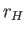from the axis of rotation, respectively. Finally, let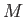be the mass of the body, andthe mass of the displaced water. It follows that the buoyancy force has an upward vertical component, and an outward horizontal component. Thus, according to standard Newtonian dynamics (Fitzpatrick 2012), the equation of horizontal motion of a general co-rotating body is(2.91)

where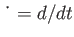. From Archimedes' principle,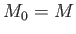for the case of a floating body that is less dense than water. However, if the body is of uniform density then, as a consequence of the curvature of the water's surface. Hence, we obtain(2.92)

In other words, a floating body drifts radially inward towards the rotation axis. On the other hand,for a fully submerged body that is more dense than water. However, if the body is of uniform density then its centers of gravity and buoyancy coincide with one another, so that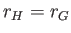. Hence, we obtain(2.93)

In other words, a fully submerged body drifts radially outward from the rotation axis. The previous analysis accounts for the common observation that objects heavier than water, such as grains of sand, tend to collect on the outer side of a bend in a fast flowing river, while floating objects, such as sticks, tend to collect on the inner side.Next: Equilibrium of a Rotating Up: Hydrostatics Previous: Curve of Buoyancy
Richard Fitzpatrick 2016-03-31